# Properties Of Multiplication - Definition with Examples

The Complete K-5 Math Learning Program Built for Your Child

• 40 Million Kids

Loved by kids and parent worldwide

• 50,000 Schools

Trusted by teachers across schools

• Comprehensive Curriculum

Aligned to Common Core

## Commutative property of multiplication

Commutative property of multiplication states that the answer remains the same when multiplying numbers, even if the order of numbers are changed. Changing the order of multiplication doesn’t change the product.

For instance,

Example 1- Let us consider two numbers 3 and 5.

On multiplying 3 lots of 5 we get, 3×5=15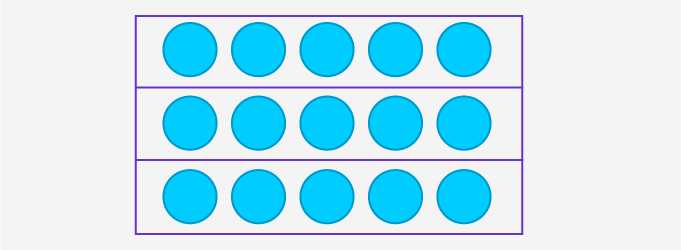Now on reversing the order of multiplication, we get 5 lots of 3

i.e. 5×3=15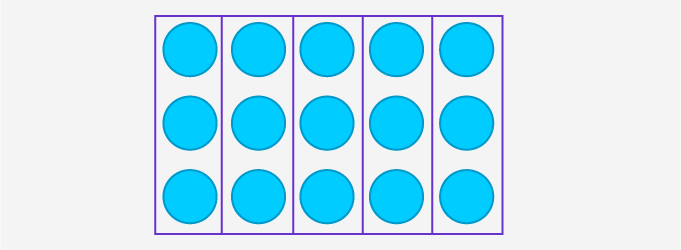As the answer is the same in both cases, we can say that multiplication is commutative.

## Associative property of multiplication

Associative property of multiplication states that if we want to multiply any three numbers together, the answer will always be the same irrespective of the order in which we multiply the numbers.

For instance,

Example 1- Let us consider any three numbers, say 2, 3, and 4 and multiply them.

Case 1: We can group the numbers as 2×(3×4)

Our answer will be: 2×(3×4)=2×12=24 [2 lots of 3 lots of 4s]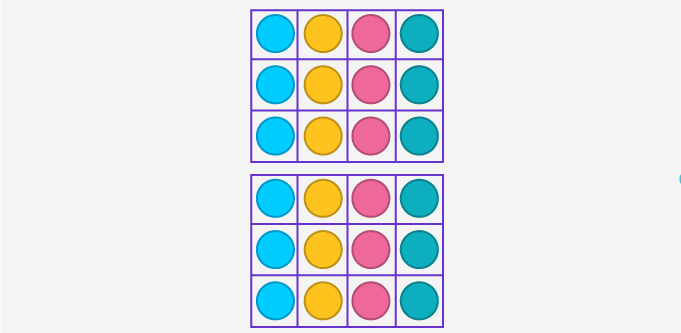Case 2: We can also group the numbers as (2×3)×4

Then our answer will be: (2×3)×4=6×4=24 [4 lots of 2 lots of 3s]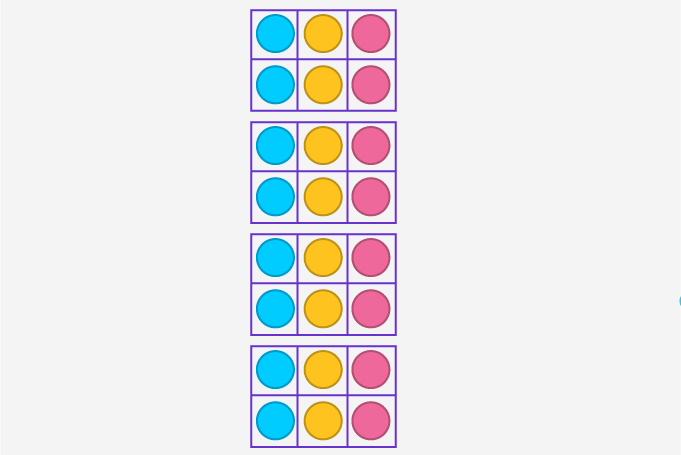As in both cases, the answer we get is the same, irrespective of the order in which the numbers are multiplied. Hence, multiplication is associative.

## Distributive property of multiplication

The distributive property of multiplication states that multiplication can be distributed over addition, as well as, subtraction.

This property helps us solve the questions with brackets. It also speeds up our mental calculations.

For instance,

Example 1- Let us consider the calculation,  2×(3+1)

Case 1: If we add first,

then our answer will be: 2×(3+1)=2×4=8 [2 lots of 4s]Case 2: If we distribute multiplication over addition,

2×(3+1)=2×3+2×1=6+2=8 [2 lots of 3s and 2 lots of 1]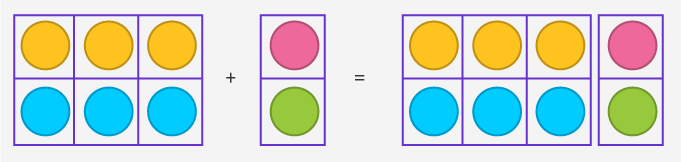As in both cases, the answer we get is the same, hence, multiplication is distributive.

## Identity property of multiplication

The identity property of multiplication states that if you multiply any number by 1, the answer will always be the same number.

For instance,

Example 1- Let us consider anyone number and multiply it by 1.

The calculation we get is 3×1=3 [3 lots of 1s]Example 2- Let us consider anyone number and multiply it by 1.

The calculation we get is 7×1=7 [7 lots of 1s]Fun Fact: If you multiply any number by 0, the answer will always be zero.

Won Numerous Awards & Honors